Fibonacci! 🌀

# G'day People!

Fourth week of school, done!
Now I can relax in a weekend drowned in homeowork! (yay?)

Anywaysssss...

# Welcome to a tutorial on The Fibonacci Sequence!

I will be covering who Leonardo Pisano Bigollo is, what one of his most famous work is about, and I've also included a quick repl where you can get as much terms (numbers) of the sequence you want! There will also be some interesting facts at the end.

All right, let's start!

## Leonardo Pisano Bigollo

Leonardo Pisano Bigollo was an Italian mathematician who lived from 1170 to 1250 (a longggg time ago!). He is best known thought as Fibonacci.

His works include of course the Fibonacci Sequence, but he also introduced to the world math concepts like the Arabic numbering system, the concept of square roots, number sequencing, and also math word problems.

## The Fibonacci Sequence

The Fibonacci Sequence, named after the mathematician who discovered it, is a series of numbers. The next number is found by adding the 2 numbers before it.

The first numbers are:

`0, 1, 1, 2, 3, 5, 8, 13, 21, 34, 55, etc.`

As you can see, the 3rd number in the sequence is `1`, made with adding `0` and `1`, the 2 numbers behind it. Then `2` is found by adding `1` and `1`. [...] `55` is found by adding `21` and `34`, and so on and so forth.

#### Golden Spiral

Now if we take the widths (the numbers) and make squares out of them, and then if we make arcs going from one corner to the other corner, we get a lovely spiral: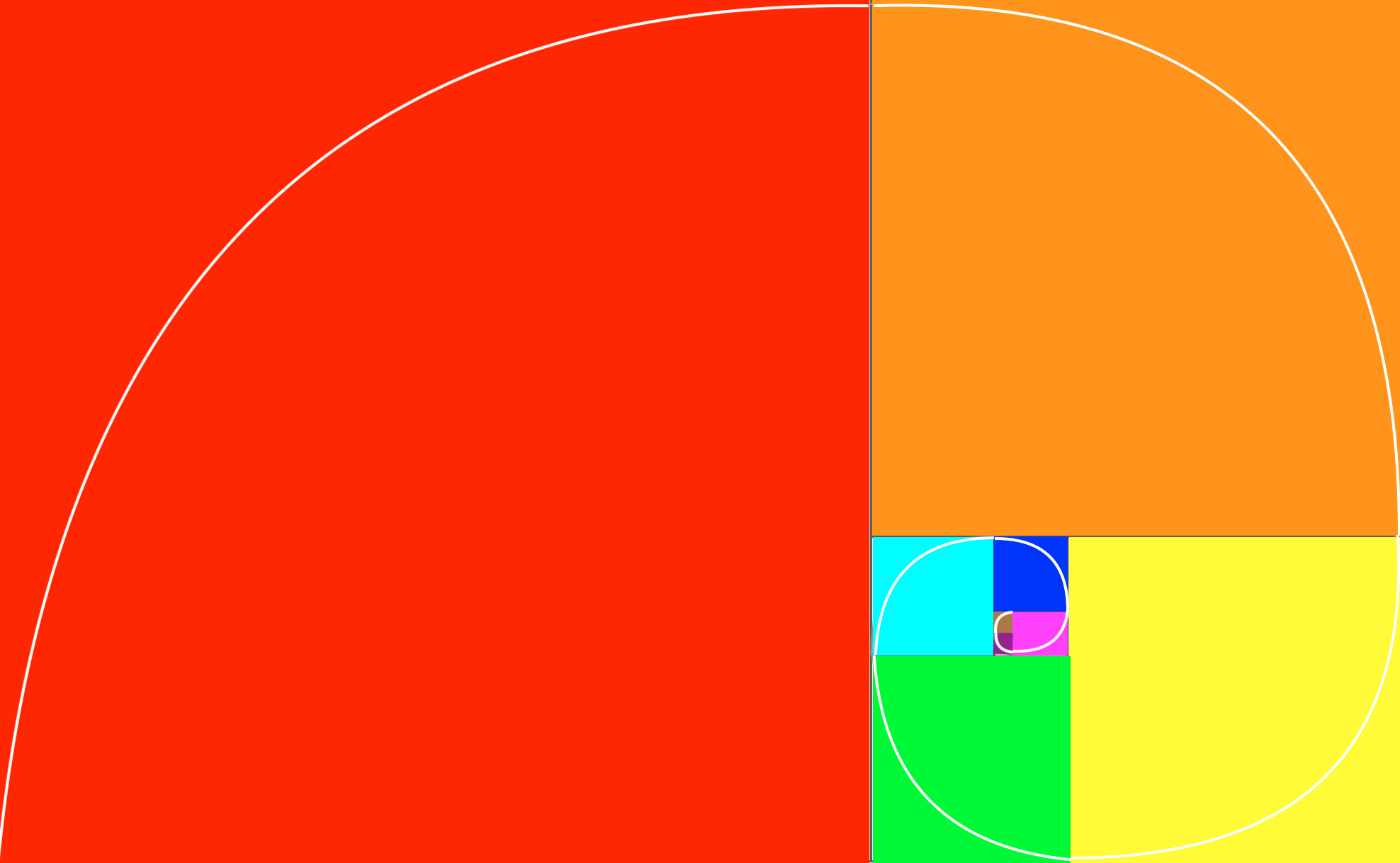As you can see, the first 2 squares in brown and purple have a width of 1, then the square in pink has a width of 2, next the square in dark blue has a width of 3, [...], and the square in red has width of of 34. Of course the loop goes on until infinty, so I havn't represented everything, but it's just to show the beginning.

###### (and sorry it's not that precise, here is a better one from google:)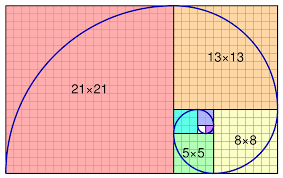The important part of this spiral is that we can find it several times in nature: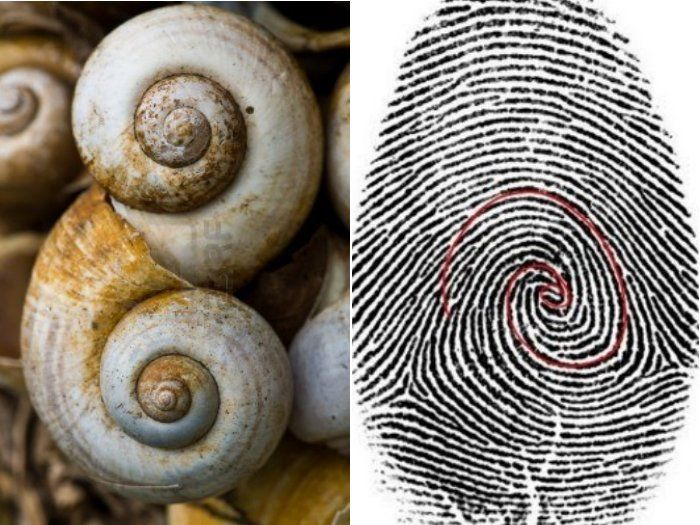This is related a bit to the Golden Ratio:

If we take the ratio of two successive Fibonacci Numbers (numbers right after each other), like say `8` and `13`, the ratios are very similar to the Golden Ratio. I'm not going to go too much into details about the Golden Ratio, but basically:

Any number that is a simple fraction (example: 0.75 is 3/4, and 0.95 is 19/20, etc) will, after a while, make a pattern of lines stacking up, which makes gaps.

(From here)

The Golden Ratio is approximately: ~1.61803...

So when we look at the ratios: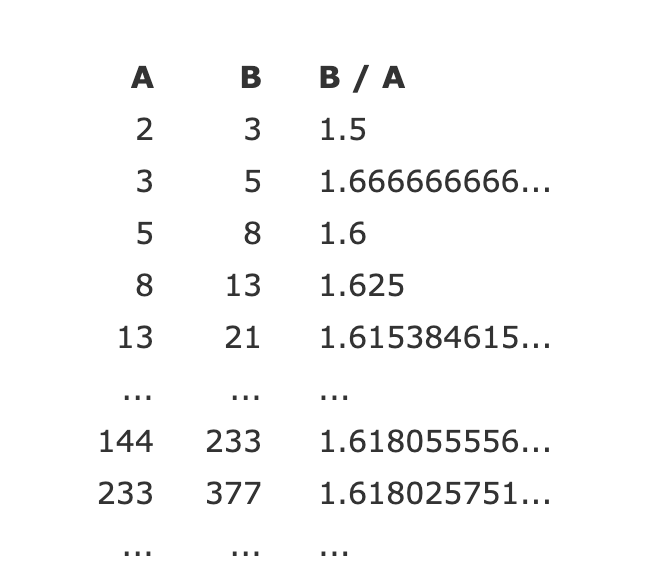The more digits the numbers have, the more the ratios look similar to the Golden Ratio!

Go here to find more out about the Golden Ratio! You'll see how similar the Fibonacci is to the Golden Ratio, especially in Nature! :)

#### Formula

First, we have to number the series from 0:

So for example term number 0 is 0, term 1 is 1, term 7 is 13, and so on.

This means that term 6 (8) = term 4 (3) + term 5 (5). We can represent it with a table with the first numbers of the Fibonacci:

So now we can write the formula: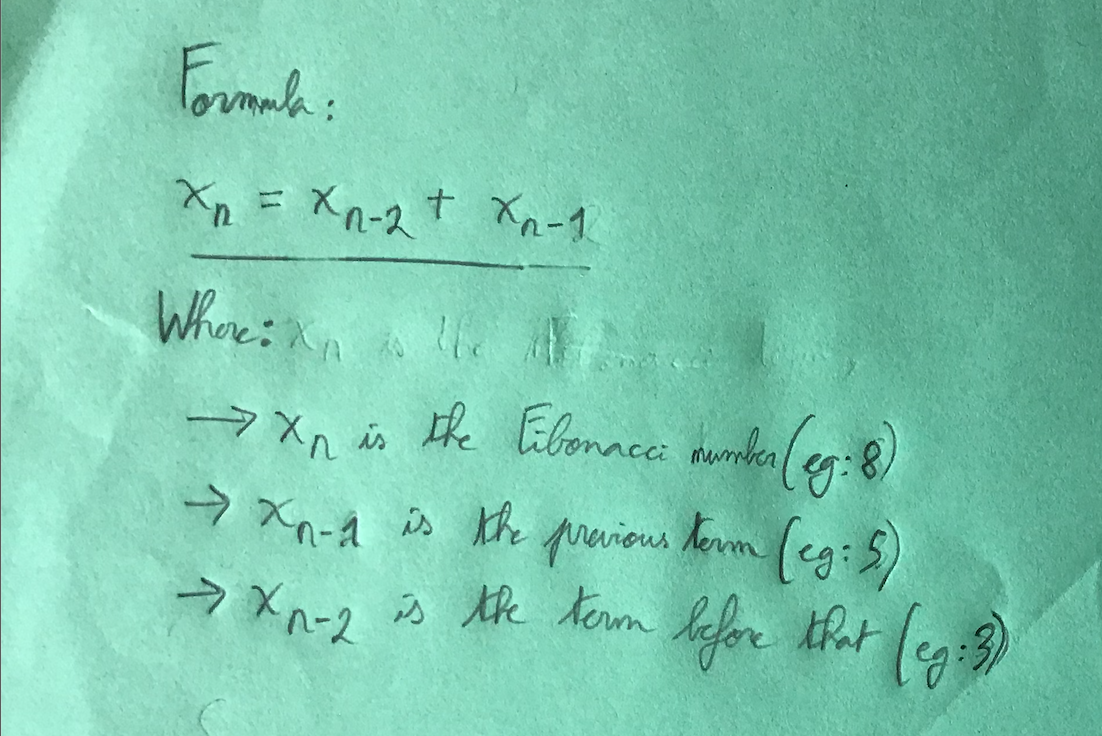Now with an example, let's calculate term 10 (which is 55):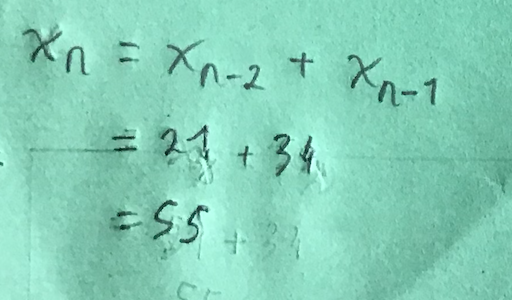## Interesting facts

#### Multiples

Every 3rd number of the Fibonacci Series is a multiple of 2: 2, 8, 34, etc.

Every 4th number of the Fibonacci Series is a multiple of 3: 3, 21, 144, etc.

Every 5th number of the Fibonacci Series is a multiple of 5: 5, 55, 610, etc.

And so on and so forth.
We can sum this up with the following: every nth is a multiple of Xn.

To visualize this better: back to the table with the numbers: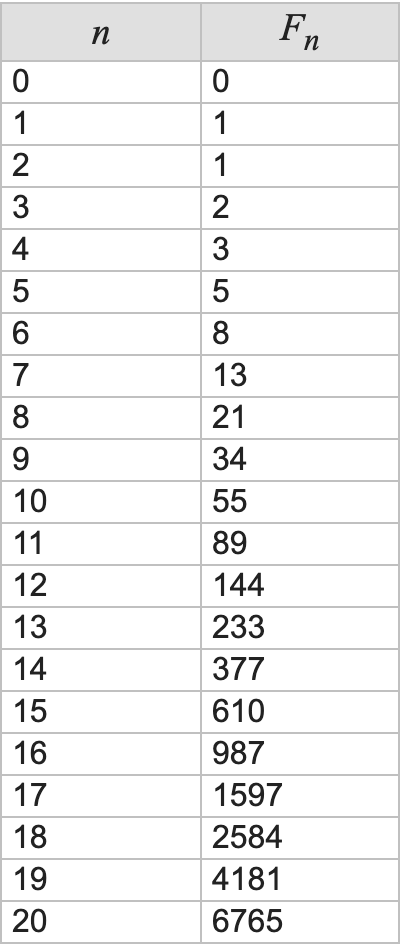As you can see, the 3rd number of the Fibonacci (starting at 1) is 2, and every 2nd number starting at 0 (2, 8, 34, etc) is a multiple of 2.

Another example:

The 5th number of the Fibonacci is 5, and every 5th number is a multiple of 5 (5, 55, 610, 6765, etc.)

#### Fibonacci Day

Fibonacci day is November 23rd; if you write like this: 11/23, we get the first 4 digits of the sequence.

#### Miles and Kilometers.

Taking the first few terms of the sequence:

`0, 1, 1, 2, 3, 4, 8, 13, 21, 34, 55, etc.`

If we take 2 consecutive numbers, we get the 1st number in miles, and the second number the equivalent in kilometers (roughly):

``````0 mi = 0 km
1 mi = 1.61 km ≈ 1
1 mi = 1.61 km ≈ 2
2 mi = 3.22 km ≈ 3
3 mi = 4.83 km ≈ 4
[...]
21 mi = 33.8 km ≈ 34
34 mi = 54.72 km ≈ 55
55 mi = 88.51 km ≈ 89
etc. ``````

As you can see, each number is the sum of the 2 numbers before it. The higher we go, the more precise it is.

#### Honeybee

This one's a bit more complex, but neverless still interesting:

A more real life, found in nature example is the ancestral lineage of the Honeybee.

In a Honeybee colony there are three types of bees:

Queen Bee — A single Female that produces eggs
Worker Bees — Females that do work and produce no eggs
Drone Bees — Males that do no work

♀ Female Honeybees are produced from the Queen’s fertilized eggs, so they have two parents, one Female and one Male

♂ Male Drone Honeybees are produced from the Queen’s unfertilized eggs, so they only have one parent, a Female

When you trace back the ancestral tree of any Honeybee (Male or Female), you’ll see that the number of bees in each prior generation matches the numbers in the Fibonacci sequence.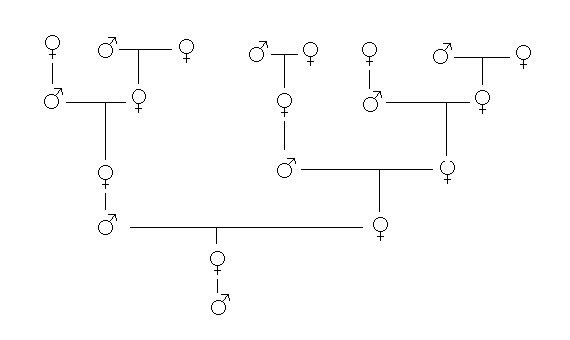(from here)

#### 1/89

`0.01123595506...`

Notice how the first 6 digits (1, 1, 2, 3, 4, 5).

However, if you write the fraction a different way:

``````0.0
0.01
0.001
0.0002
0.00003
0.000005
0.0000008
0.000000013
0.0000000021
0.00000000033
etc.``````

You see that the numbers at the end are the terms of the Fibonacci. However, they're overlapping; if you add those numbers above, you'll get an approximation of `1/89` (something like 0.011235955056179775.), and the more deeper you go, the more precise result you'll get, and therefore the more Fibonacci terms you'll get.

### The Program

#### Output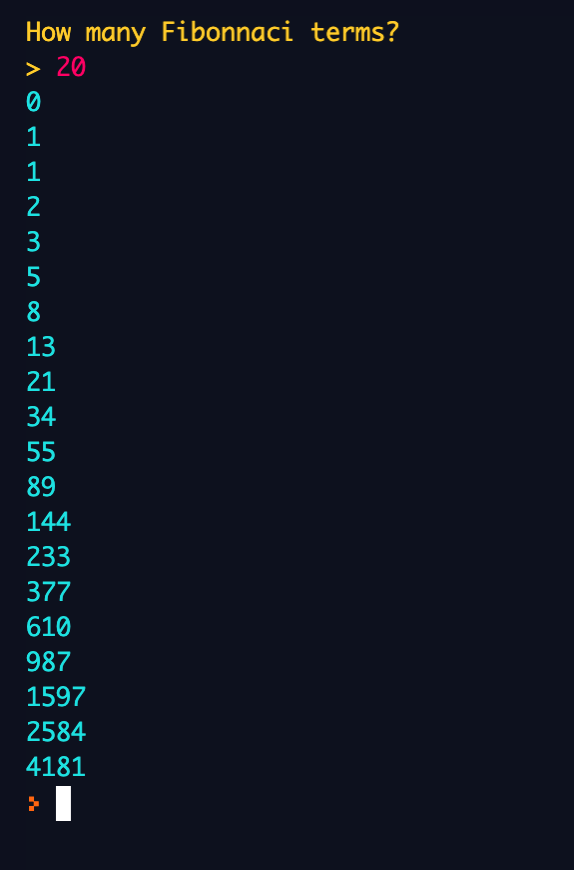So the program will ask you how many Fibonacci terms you want. Remember that if you enter a value too big, it'll take a pretty long time and it might crash lol.

#### Code

The code is pretty straightforward, I also added comments so you can understand it better. I have the basic code, but also added some colors, and also a `try` and `except` in case the user types something else than integers, and also a `while True` loop so that it loops forever.

First there's two variables `x1` and `x2` that have the initial value of `0` and `1`, respectively. Then there's an `input` to ask the user how many terms they want. Next there's a `for` loop that repeats for the number of times the user said before.

Afterwards, there's a `print` statement that outputs the Fibonacci term (with color). Then, it makes a new variable called `next_x` which is the sum of the previous terms, `x1` and `x2`, like in the Fibonacci sequence. And then, `x1` becomes `x2`, and `x2` becomes `next_x`. This is to kind of "advance" the numbers; put all the numbers forward.

Here my sources:

All right, well, guess that's all lol! Hope you've learnt something and enjoyed the beautiful colors of the repl!

Now you can impress all your friends, family, and math teachers with these amazing facts!

🌀 🌀 🌀 🌀

# Everyone have a super-duper day!

Cya next time! :D

You are viewing a single comment. View All
DannyIsCoding (691)